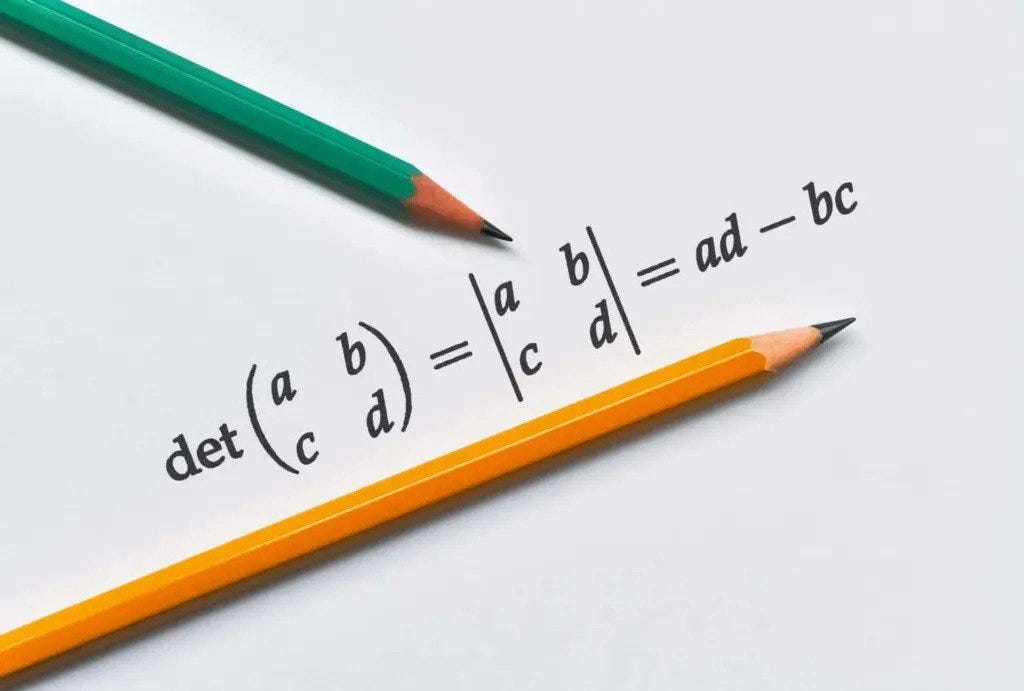# Determinant of a Matrix without NumpyDeterminant of a matrix.

Finding the determinant of a 2x2 matrix or even a 3x3 matrix can be easy enough for us to do it on a piece of paper. But as the size of the matrix increases it becomes exponentially hard for us to find it manually and because of this we seek the help of computer to do it for us.

Coding helps us to do any task if the procedure to follow for that process is well defined. And it is the same for us. Finding the determinant of a matrix is also a well defined process. If you are using python and numpy then it is as simple as writing a single function to get the determinant of any size matrices. But doing so we wouldn’t learn the working of the code or even the procedure itself, so here I am trying find the determinant of a matrix using python with out using the numpy library. We will be trying to do it from scratch.

If you don’t know the basic method for finding the determinant of a matrix, kindly go through following page before reading the rest of this article.

# CODE and EXPLANATION

Before going through the code let me give you flavor of how I have represented matrices in this code. I have used a list of lists; essentially, each of the row is a list in itself. One example is given below:

`[[1,2,4], [3,4,7], [5,6,7]]`

Next step for us is to use the only library we would be using in this, that is “ copy ” library. This is only to to create a perfect copy of the matrix itself and nothing more.

`import copy`

Next we define the functions we will be using to find the determinant of a matrix.

The new_matrix() function takes in an array and a number ‘i’. It then creates a copy of the array and then removes the first row and then from each of the remaining rows removes the ith element and returns the resultant array. If the length of the copied array is just 2 then it would simply return the array itself without dropping anything.

`def new_matrix(a,i):#FUNCTION TO FIND THE NEW MATRIX      arr = copy.deepcopy(a)    arr = a[:]    if len(arr) == 2:        return arr    else:        arr.pop(0)        for j in arr:            j.pop(i)                    return arr`

The determinant() function is the main function that runs the program and help us to find the determinant of an array. It takes in an array, and if the length of the array is 1, then it would return the only element in that array.

If the passed array has a length of 2, then the base condition of our recursive function kicks in and it returns the answer of the following equation :

pro = a*a — a*a

If the passed array has a length greater than 2, then it would initialize the pro variable as 0 and iterate through the length of the first row, and find the value of ((-1)**i)*a[i]*determinant(new_matrix(a,i)) and adds it it pro. In each of the iteration, the value produced is the product of the ith element of the first row and the returned value of the determinant() function in which the new_matrix() function is called passing the array and the iteration variable i. This is basically the iteration part of the whole process. The determinant function is called by itself(recursion) until the matrix being passed inside the new_matrix function is a 2x2 matrix. After all of that it is multiplied with either -1 or 1. If i is odd then the value is multiplied with -1, and if it is even it will be multiplied with 1.

After each of the iteration, the value obtained is added to the pro variable.

`def determinant(a):#FUNCTION TO FIND THE DETERMINANT OF A MATRIX    if len(a) == 1:        pro = a        return proelif len(a) == 2:        pro = a*a - a*a        return pro            else:        pro = 0        for i in range(len(a)):            pro += ((-1)**i)*a[i]*determinant(new_matrix(a,i))            return pro`

Testing our code with some matrixes of different sizes to see if it works alright.

`A = B = [[1,2,4],[3,4,7],[5,6,7]]C = [[1,2,3,4],[4,3,5,6],[8,4,2,1],[3,2,4,1]]D = [[1,3,5,7,9],[4,6,3,7,5],[5,10,8,3,1],[1,5,3,7,6],[8,1,7,5,8]]print("The determinant of A --> ",determinant(A))print("The determinant of B --> ",determinant(B))print("The determinant of C --> ",determinant(C))print("The determinant of D --> ",determinant(D))`

OUTPUT obtained

`The determinant of A -->  2The determinant of B -->  6The determinant of C -->  -99The determinant of D -->  -687`

The code in whole is provided below:

`import copydef new_matrix(a,i):#FUNCTION TO FIND THE NEW MATRIXarr = copy.deepcopy(a)     if len(arr) == 2:                return arr    else:        arr.pop(0)        for j in arr:            j.pop(i)                    return arr    def determinant(a):#FUNCTION TO FIND THE DETERMINANT OF A MATRIX    if len(a) == 1:        pro = a        return proelif len(a) == 2:        pro = a*a - a*a        return pro            else:        pro = 0        for i in range(len(a)):            pro += ((-1)**i)*a[i]*determinant(new_matrix(a,i))            return pro                     A = B = [[1,2,4],[3,4,7],[5,6,7]]C = [[1,2,3,4],[4,3,5,6],[8,4,2,1],[3,2,4,1]]D = [[1,3,5,7,9],[4,6,3,7,5],[5,10,8,3,1],[1,5,3,7,6],[8,1,7,5,8]]print("The determinant of A --> ",determinant(A))print("The determinant of B --> ",determinant(B))print("The determinant of C --> ",determinant(C))print("The determinant of D --> ",determinant(D))`

Thank you guys for being patient and going through my humble article, if you guys have any doubt please feel free to leave a response.

Masters student at IIITM - Kerala

## More from Amal R

Masters student at IIITM - Kerala